# A POSTERIORI ERROR ESTIMATES FOR FINITE ELEMENT DISCRETIZATIONS OF PARABOLIC OPTIMAL CONTROL PROBLEMS

N/A
N/A
Protected

Share "A POSTERIORI ERROR ESTIMATES FOR FINITE ELEMENT DISCRETIZATIONS OF PARABOLIC OPTIMAL CONTROL PROBLEMS"

Copied!
237
0
0

Full text

E4,3n ,Eb4,3n Co-state variable spatial error estimator for POCP with control acting on LDMF. E4,4n , Eb4,4n Co-state variable temporal error estimator for POCP with control operating in LDMF.

## Description of the problems

Note that the control variable u appears in the Neumann boundary condition, and nE is the unit outwardly normal to the boundary. The shape's border control problem is generally considered more physically realistic because the control is only applied through the border.

## Notations and preliminaries

In particular, for p= 2 and q = 2, the above inequality is known as the Cauchy-Schwarz inequality in Rd. Then f is said to be Gˆateaux differentiable at x∈X˜0, and T is referred to as the Gˆateaux derivative of f at x.

## Background and motivation

In this work, we treat different a posteriori error estimates for the control problem for different dimensions of the manifold. We have proved different types of a posteriori error estimates for the state variable at the norm L2(0, T;L2(Ω)) while the error for the control variable at the norm L2(0, T;Rm) or L2( 0, T;L2(γ(t)))-norm according to the dimension of manifolds.

## Organization of the thesis

We derive a posterior upper bound for the state, co-state, and control variables on the norm L∞(0, T;L∞(Ω)). We derive a posterior estimate of the error for the state variable at the norm L2(0, T;L2(Ω)) while the error for the control variable is set at the norm L2(0, T;Rm) or L2(0, T;L2( γ(t)))- norm according to the dimension of the collector γ(t).

## Discrete optimal control problem

Representation of the bilinear form: For a function vh ∈Vhn(0≤n≤N), the bilinear form a(vh, w) can be represented as. Forvh ∈Vhn, let Aelvh be the regular part of the ∆vh distribution, which is defined as a piecewise continuous function such that.

## A posteriori error analysis

To estimate the term ζn1, which measures the spatial error and the lattice shift, we will exploit the orthogonality property of the elliptic reconstruction definition (2.31). A similar argument as in the case of the state variable and the use of the interpolation operator ˆΠn defined as in Proposition 2.3.2 leads to.

## Numerical assessments

Numerical results on uniform and adaptive grids at finite time T = 1.0 are listed in Table 2.1. We further observe that the mesh moves away from the surroundings at a constant speed at t = 0.5, but the shape of the solution remains unchanged. Figure 2.6 shows that the network adapts very well near the discontinuous line x1 +x2 = 1.

It is clear from Table 2.2 that the adaptive mesh generated via the error indicators produces promising numerical results.

## Concluding remarks

1585-1594] in combination with heat kernel estimates for linear parabolic problems to derive a posteriori error estimates for the state, co-state and control variables in the L∞(0, T;L∞(Ω)) norm. To limit some of our fully discrete a posteriori error estimates of the form Φ1 − Φ2−(Φh1−Φh2), where Φh1 and Φh2 are related to different finite element spaces defined on meshes in adjacent time steps, we recall the following results from [21 ]. We derive a posteriori error estimates for the state, co-state and control variables in the L∞(0, T; L∞(Ω)) norm for both the semidiscrete and fully discrete variation discretization approaches.

In Section 3.2 we discuss variational discretization approximation for the control problem and derive a posteriori error estimates for the semidiscrete problem.

## Analysis for semidiscrete control problem

To begin with, we first establish some intermediate error estimates for the state and co-state variables in the L∞(0, T;L∞(Ω)) norm that allow us to prove the main results of this section. As a result of the elliptic error estimation in Lemma 3.1.1, we obtain the following bounds for the elliptic reconstruction errors. The proof of the second inequality can be treated in a similar way with the fact that ˆξp(T) = 0.

To derive posterior error bounds for the state and co-state variables, we decompose the errors as follows: 3.38).

## Analysis for fully discrete control problem

As in the case of semi-discrete error analysis, we first derive some intermediate error estimates for the state and co-state variables in the L∞(0, T;L∞(Ω)) norm. L∞(L∞)−A posteriori error estimates for POCP 74 Lemma 3.3.2 (Parabolic error estimates for the state and co-state variables). hK, the following estimates apply:. To derive a posteriori error bounds for the state and co-state variables, we decompose the errors as follows:.

In the following lemma, we derive the a posteriori error estimate for the control variable in the norm L2(0, T;L2(Ω)).

## Numerical assessments

In the last step, if the time error estimator is much smaller than the prescribed time tolerance time, we increase the time step size by multiplying a factor of δ2. In Figures 3.2 and 3.3, the approximate solutions of y and u are plotted on uniform mesh, adaptive mesh step-(I) and adaptive mesh step-(II), respectively, at the end time T = 1.0. Figure 3.1 clearly shows that the mesh adapts very well near the line x1+x2 = 1 where the solution is discontinuous.

L∞(L∞)−A posteriori error estimates for POCP 85 Table 3.1: Comparison of uniform mesh, adaptive mesh step-(I) and adaptive mesh step-(II) data for the initial mesh N = 180.

## Concluding remarks

This chapter deals with spatiotemporal analysis, a posteriori error analysis with finite element approximation of POCP where the control acts on a lower dimensional manifold. The manifold considered in this chapter can be a point, a curve, or a surface which lies completely in the domain of space. Further, the manifold is assumed to be either time-independent or time-evolved.

The spatial discretization consists of semi-linear and continuous finite elements for the state and covariates, and semi-constant functions are used to approximate the control variable.

## Introduction

The following theorem represents the correctness of the solution to problem (4.2) in different dimensions of the multiplicity γ(t). They derived a priori estimates of the error for the state variable in the L2(Ω)-norm, while the error for the control variable in the U-norm is U = L2(γ(t)) or U = Rm depending on the manifold dimension. This chapter attempts to study a posteriori error analysis of fully discrete finite element approximations to the state, covariance, and control variables for control problems with controllers acting on a low-dimensional manifold.

We derive a posteriori error estimates for the state variable in the L2(0, T;L2(Ω))-norm, while the error for the control variable in L2(0, T;Rm) or L2(0, T; L2(γ(t )))-norm according to the dimension of the manifold.

## Discrete optimal control problem

A posteriori error estimates for the state, co-state and control variables are derived in Section 4.3. Controls Acting on Lower Dimensional Manifolds 94 Then the fully discrete finite element approximation of the optimal control problem is to seek a pair (yhn, unh)∈Vhn×Uad,hn , for n= 1, 2,.

## A posteriori error analysis

Controllers Acting on Low-Dimensional Manifolds 96 Before developing the error analysis, we collect some stability results for the state variable. Controls acting on lower-dimensional manifolds 103 The expression I9 derives from the definition of the L2-projection operator Ln and Lemma 1.2.3. Let γ(t) be an r-dimensional manifold lying entirely inside Ω with d−r > 1 when r = 1 and d = 3. Controls Acting on Lower-Dimensional Manifolds 105 Proof.

Controls Acting on Lower-Dimensional Manifolds 106 By arguments similar to the previous example (see the proof of (4.47) in Theorem 4.3.1, for d−r= 1, r≥1) and using the regularity result (4.63), it is easy to obtain.

## Numerical assessments

Controllers acting on low-dimensional dividers 115 from the prescribed time tolerance, we then increase the time step size by multiplying the factor δ2. The first case deals with the optimal control problem with a low-dimensional manifold as a moving point γ(t) = (γ1(t), γ2(t)), while the second case deals with a moving interval. For spatial discretization, we use piecewise linear and continuous finite elements for the state and state variables, and piecewise constant functions for the control variable.

Controls Acting on Lower-Dimensional Manifolds 119 Table 4.2: Error estimates for the state and covariance variables in the norm L2(0, T;L2(Ω))- and the control error in L2(0, T;L2( γ(t))) -norm, on uniform network, adaptive network Step-(I) and adaptive network Step-(II).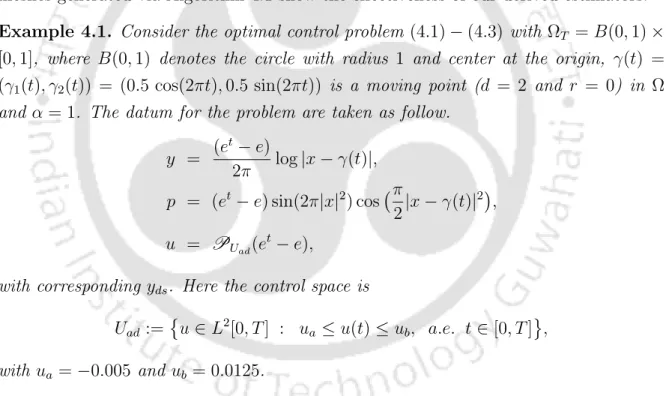Table 4.1 shows the mesh information with error estimates for the state and con- con-trol variable in the L 2 (0, T ; L 2 (Ω)) and L 2 (0, T ; R m ) norms, respectively, on the uni-form mesh, adaptive mesh-(I) and adaptive mesh-(II)

## Concluding remarks

Local a posteriori error estimates for PBCP 127. The last case deals with control problem with observation of the final state y(x, T). They have obtained a posteriori error estimates for the co-state and control variables in the L2(Γ) norm for both observations, while the error bound for the state variable is proven in the L2(Γ) norm or L2(Ω) ) norm according to the observation spaces. We derive locala posteriori upper bounds for the state, the co-state, and the control variables in the L2(0, T;B) norm for observing the limit state as well as for the final state.

Furthermore, the posterior error estimate of the state variable for the distributed state observation is proved in the L2(0, T;L2(Ω))-norm, while the error bounds for the state and control variables are in the L2( 0, T;B)-norm .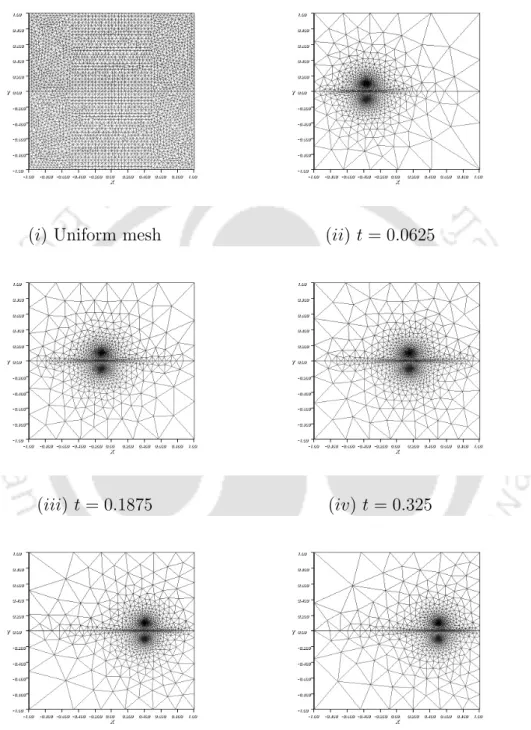Figure 4.4: Uniform mesh (i) and Adaptive meshes (ii) − (vi) (at Step-(II)) at different time levels t = 0.0625, 0.1875, 0.325, 0.450, 0.500, for Example 4.2.

## Discrete optimal control problems

Local a posteriori error estimates for PBCP 132 For the purpose of deriving local a posteriori error estimates, we consider the set. To understand the triangulations ThnandTΩnl, a typical discretization of Ω⊂R2 is shown in Figure 5.1 (a) and (b). We now recall some important interpolation approximation properties and trace result in the following lemmas.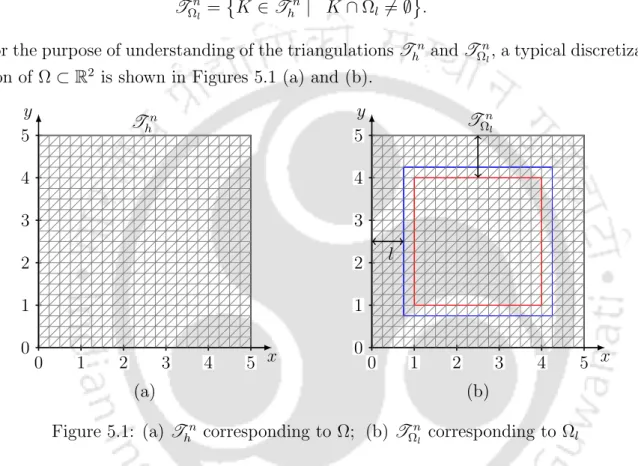Figure 5.1: (a) T h n corresponding to Ω; (b) T Ω n l corresponding to Ω l

## A posteriori error analysis

Local a posteriori error estimates for PBCP 134 exist a positive constant C5,2 and wh ∈Uad,hn such that. Local a posteriori error estimates for PBCP 135 In view of (5.43), an application of Young's inequality leads to the bound for J1,. 5.45) To boundJ2 we use the Cauchy-Schwarz inequality and the Young's inequality to have. Local a posteriori error estimates for PBCP 145 Combining the above estimates with (5.63) and using the constraint property of the bilinear form, we conclude that.

Local a posteriori error estimates for the PBCP 148 control variable derived from the variational inequality.

Let the assumptions of Lemma 5.3.4 hold and let g0(·) be a continuous Lipschitz function in a neighborhood of y with observation space Osp2. Local a posteriori error estimates for PBCP 150 In the following lemma, we provide an intermediate posterior error estimate for the state variable. Let the assumptions of Lemma 5.3.4 be satisfied and let g0(·) be a continuous Lipschitz function in a neighborhood of y with observation space Osp2.

Suppose the assumptions of Lemma 5.3.4 are satisfied, and let g0(·) be a local Lipschitz continuous function in a neighborhood of y with observation space Osp2.

The constant C5,19 depends on α0, α1, the convexity constant C5,1, the Lipschitz constant CL, the bound CM of the cut-off function, the regularity constant CR, and the constants C˜I,j, j = 1,. The constant C5,22 depends on α0, α1, the Lipschitz constant CL, the boundCM of the cut-off function, the regularity constant CR and the constants C˜I,j, j = 1,. By the continuity of the bilinear form and the Young's inequality we have for the term (III)2,.

The constant C5,25 depends on α0, α1, the Lipschitz constant CL, the limit CM of the truncation function, the stability constant CR, the constant C5,21 defined in Lemma 5.3.7, and the constants C˜I,j, j = 1,.

## Numerical assessments

Local a posteriori error estimates for PBCP 159 Table 5.1: Comparison of results on uniform grid Thn and adaptive grid with l = 0.1 and tolerance = 10−4. Local a posteriori error estimates for PBCP 160 Table 5.3: Mesh information and values ​​of the leading terms of the estimators at TΩnl with l = 0.2. From Tables 5.6−5.8, we note that the values ​​of the leading terms decrease as the distance l decreases.

Tables 5.10-5.12 show the values ​​of the leading terms revealing the effectiveness of the estimators.

## Concluding remarks

Local a posteriori error estimates for PBCP 164 Table 5.9: Comparison of results on uniform grid Thn and adaptive grid with l = 0.1 and tolerance = 10−5. Local a posteriori error estimates for PBCP 165 Table 5.11: Grid information and leading term values ​​of the estimators at TΩnl with l = 0.2. Local a posteriori error estimates for PBCP 166 for state and covariance approximations.

Assume that the pair (y∗, u∗) conforms to both the necessary first-order optimality condition (6.7) and the constraints (6.3) of the boundary control problem.

## Discrete optimal control problem and stability results

Local a posteriori error estimates for nonlinear PBCP 171 As a result of the above Theorems, the following theorem states that the optimization problem (6.6) has a solution. Earlier, Liu and Yan  derived locala posteriori error estimates for finite element approximation of elliptic boundary control problems using the duality argument. Since in many applications it is often useful to study the behavior of the state and co-state variables in a small neighborhood of the boundary, an attempt has been made in this chapter to obtain local a posteriori error estimates for the nonlinear PBCP in a bounded convex domain.

The local a posteriori error estimates for fully discrete nonlinear PBCP are performed in Section 6.3.

A posteriori error analysis

Numerical assessments

Concluding remarks

Extensions and remarks

Figure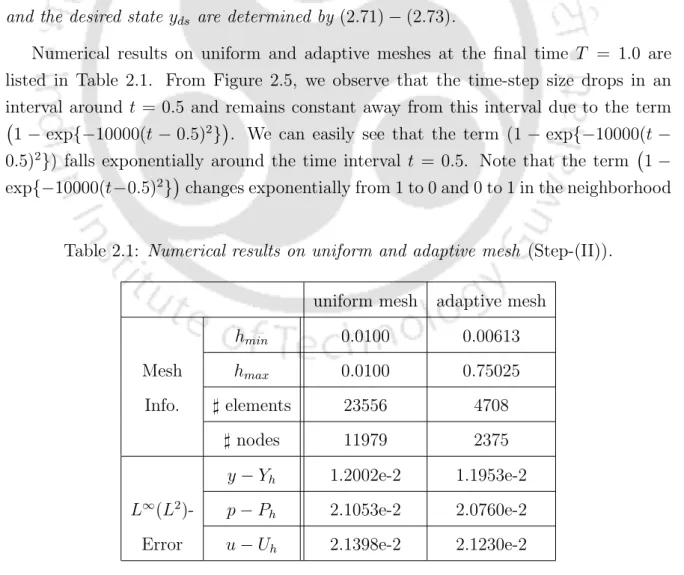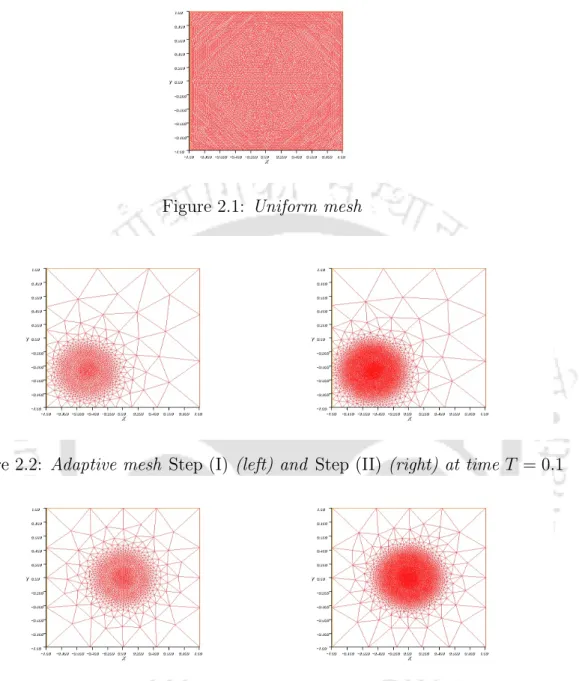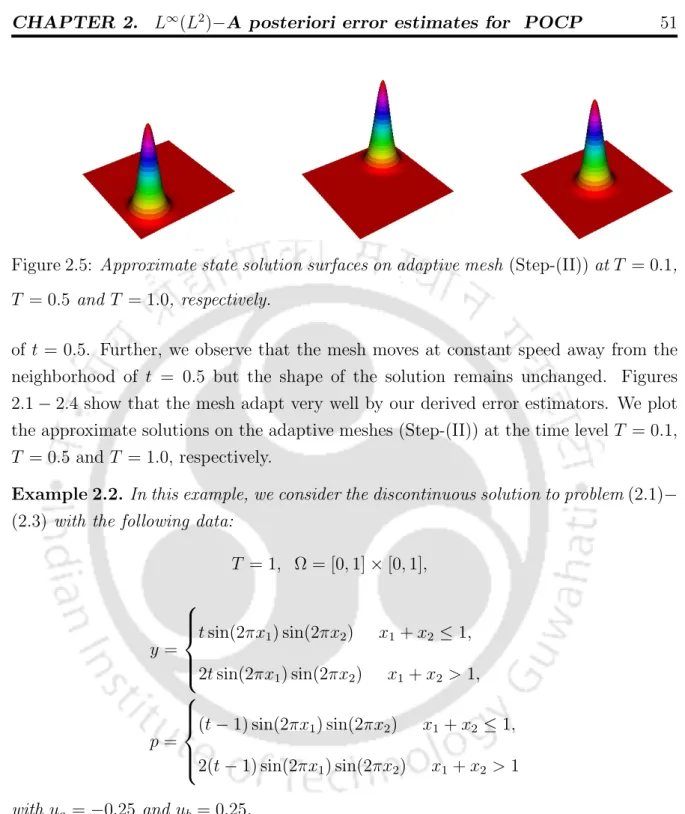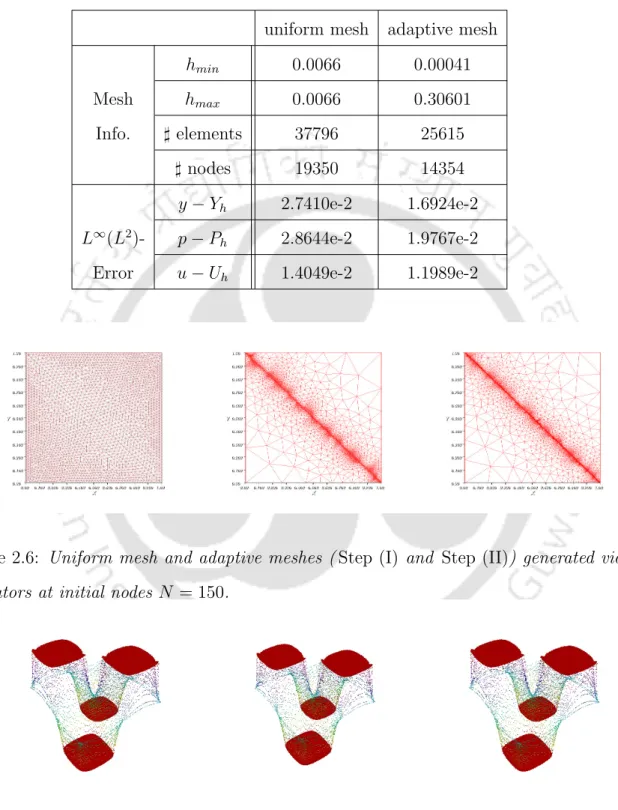+7

References

Related documents

12.2 The documentary evidence of the Bidder’s qualifications to perform the contract if its bid is accepted shall establish to the Purchaser’s satisfaction: a that, if required in the

Proceedings of ISSS 2008 International Conference on Smart Materials Structures and Systems July 24-26, 2008, Bangalore, India ISSS-2008/P98 An Ultra-Stable Cu Nanowire under

Conclusion Among the β-prism I fold lectins of known three- dimensional structure, all except banana and grifﬁ thsin lectin carry one carbohydrate-binding site per domain/ subunit;

The MCGL method is able to model the decision making process of individuals under the framework of MCDA, by revising some of the MCDM mainstream postulates and practices in order to

A.J.INSTITUTE OF MEDICAL SCIENCES & RESEARCH CENTRE DEPARTMENT OF GENERAL SURGERY Name: Dr.Kalpana Shridhar Department: General Surgery Department Designation: Professor

Giri Institute of Labour http://www.indialabourarchives.org/sources/ jnu.htm • Indian Institute of Science NCSI http://vidya-mapak.ncsi.iisc.ernet.in/cgi-bin/library • Indian

Retail salespeoples’ views of work related situations Situation Percentage of respondents who definitely believe situation poses an ethical problem Percentage respondents

A.J.INSTITUTE OF MEDICAL SCIENCES & RESEARCH CENTRE DEPARTMENT OF GENERAL SURGERY Name: Dr.SUHAS S.KUMAR Department: General Surgery Department Designation: Assistant Professor

2.4 Various E-learning platforms:- During the covid-19 pandemic situation learning has been very popular such as using various online application for teaching learning methods example